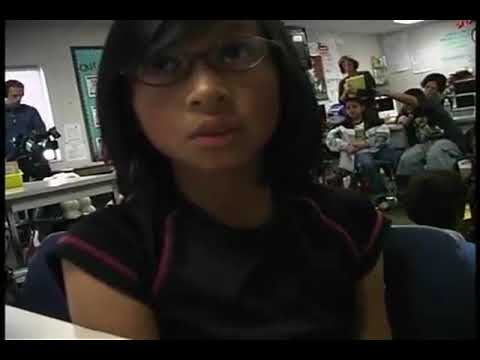COVID-19 presents an unprecedented challenge for teachers, students, and parents. Explore resources from IBM and partners here.
Video

# Multiplying 3-digit by 1-digit (Full video)

Khan Academy

## Supporting grades: 4

Description: Learn to multiply a 3-digit number by a 1-digit number without regrouping. In this video, we will multiply 4x201. Created by Sal Khan. Let's multiply 4 times 201 just to simplify things a little bit. Now, just like we did when we were multiplying a one digit times a two digit, we do essentially the same process. So we put a 4 right over there in the ones place.

You must log inorsign upif you want to:*

*Teacher Advisor is 100% free.

### Other videos you might be interested in### Number Operations: Multiplication (Full video)

#### Inside Mathematics